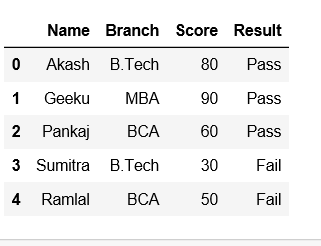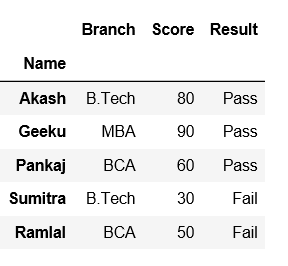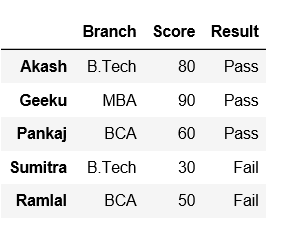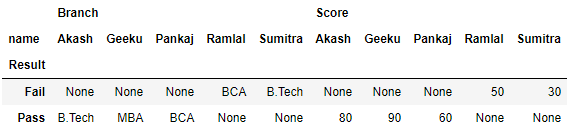Related Articles

# How to Convert Dataframe column into an index in Python-Pandas?

• Last Updated : 21 Jul, 2021

Pandas provide a convenient way to handle data and its transformation. Let’s see how can we convert a data frame column to row name or index in Pandas.
Create a dataframe first with dict of lists.

## Python3

 `# importing pandas as pd``import` `pandas as pd` `# Creating a dict of lists``data ``=` `{``'Name'``:[``"Akash"``, ``"Geeku"``, ``"Pankaj"``, ``"Sumitra"``, ``"Ramlal"``],``       ``'Branch'``:[``"B.Tech"``, ``"MBA"``, ``"BCA"``, ``"B.Tech"``, ``"BCA"``],``       ``'Score'``:[``"80"``, ``"90"``, ``"60"``, ``"30"``, ``"50"``],``       ``'Result'``: [``"Pass"``, ``"Pass"``, ``"Pass"``, ``"Fail"``, ``"Fail"``]}` `# creating a dataframe``df ``=` `pd.DataFrame(data)`` ` `df`

Output:Method #1: Using set_index() method.

## Python3

 `# importing pandas as pd``import` `pandas as pd` `# Creating a dict of lists``data ``=` `{``'Name'``:[``"Akash"``, ``"Geeku"``, ``"Pankaj"``, ``"Sumitra"``, ``"Ramlal"``],``       ``'Branch'``:[``"B.Tech"``, ``"MBA"``, ``"BCA"``, ``"B.Tech"``, ``"BCA"``],``       ``'Score'``:[``"80"``, ``"90"``, ``"60"``, ``"30"``, ``"50"``],``       ``'Result'``: [``"Pass"``, ``"Pass"``, ``"Pass"``, ``"Fail"``, ``"Fail"``]}` `# Creating a dataframe``df ``=` `pd.DataFrame(data)` `# Using set_index() method on 'Name' column``df ``=` `df.set_index(``'Name'``)` `df`

Output:Now, set index name as None.

## Python3

 `# set the index to 'None' via its name property``df.index.names ``=` `[``None``]` `df`

Output:Method #2: Using pivot() method.
In order to convert a column to row name or index in dataframe, Pandas has a built-in function Pivot. Now, let’s say we want Result to be the rows/index, and columns be name in our dataframe, to achieve this pandas has provided a method called Pivot. Let us see how it works,

## Python3

 `# importing pandas as pd``import` `pandas as pd` `# Creating a dict of lists``data ``=` `{``'name'``:[``"Akash"``, ``"Geeku"``, ``"Pankaj"``, ``"Sumitra"``, ``"Ramlal"``],``       ``'Branch'``:[``"B.Tech"``, ``"MBA"``, ``"BCA"``, ``"B.Tech"``, ``"BCA"``],``       ``'Score'``:[``"80"``, ``"90"``, ``"60"``, ``"30"``, ``"50"``],``       ``'Result'``: [``"Pass"``, ``"Pass"``, ``"Pass"``, ``"Fail"``, ``"Fail"``]}` `df ``=` `pd.DataFrame(data)` `# pivoting the dataframe``df.pivot(index ``=``'Result'``, columns ``=``'name'``)` `df`

Output:Attention geek! Strengthen your foundations with the Python Programming Foundation Course and learn the basics.

To begin with, your interview preparations Enhance your Data Structures concepts with the Python DS Course. And to begin with your Machine Learning Journey, join the Machine Learning – Basic Level Course

My Personal Notes arrow_drop_up